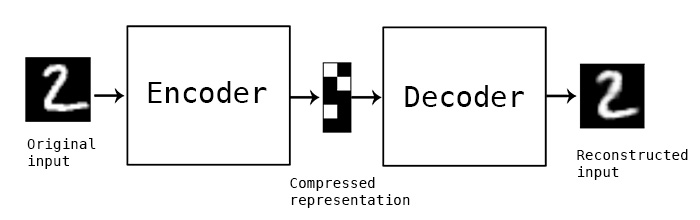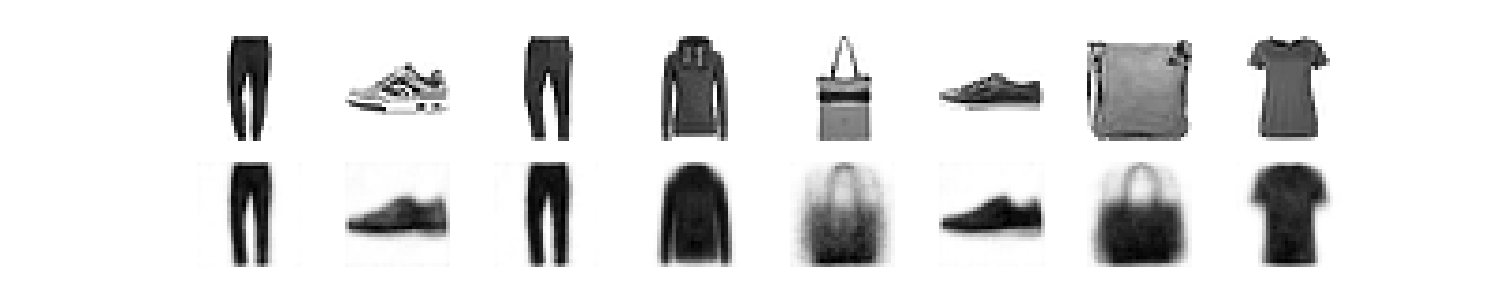# What are Autoencoders?(Image source Keras)

An autoencoder is a neural network that is trained to attempt to copy its input to its output. Internally, it has a hidden layer h that describes a code used to represent the input. The network may be viewed as consisting of two parts: an `encoder` function h=f(x) and a `decoder` that produces a reconstruction r=g(h). If an autoencoder succeeds in simply learning to set g(f(x)) = x everywhere, then it is not especially useful. Instead, autoencoders are designed to be unable to learn to copy perfectly. Usually they are restricted in ways that allow them to copy only approximately, and to copy only input that resembles the training data. Because the model is forced to prioritize which aspects of the input should be copied, it often learns useful properties of the data. 

## What are Variational Autoencoders?

Variational Autoencoders extend the core concept of Autoencoders by placing constraints on how the identity map is learned. These constraints result in VAEs characterizing the lower-dimensional space, called the latent space, well enough that they are useful for data generation. VAEs characterize the latent space as a landscape of salient features seen in the training data, rather than as a simple embedding space for data as AEs do.(The first line shows the input images, and the second presents the ones generated by the autoencoder.)

This project introduces an example of a `convolutional (variational) autoencoder` that reads an input image, encodes the image into a lower dimensional latent representation, then decodes the latent representation to reconstruct the imput image. The autoencoder is implemented using the Keras, and it is based on convolutional neural networks leveraging Conv2D layers in the `encoder`, and Conv2DTranspose layers in the `decoder`. The autoencoder is trained using the `Fashion-MNIST` dataset. Each image in this dataset is 28x28 pixels. For this reason, the input shape of the `encoder` was set to `(28, 28, 1)` as well as for the output shape of the `decoder`.

## The Fashion-MNIST Dataset

`Fashion-MNIST` is a dataset of Zalando ‘s article images—consisting of a training set of 60,000 examples and a test set of 10,000 examples. Each example is a 28x28 grayscale image, associated with a label from 10 classes. `Fashion-MNIST` is intended to serve as a direct drop-in replacement for the original MNIST dataset for benchmarking machine learning algorithms. It shares the same image size and structure of training and testing splits. 

## Scripts

• `conv_autoencoder.py`: contains the implementation of a Convolutional Autoencoder and a Convolutional Variational Autoencoder.

• `train_conv_autoencoder.py`: trains the selected autoencoder model on the Fashion MNIST dataset, and saves the weights and parameters of the model under the selected directory (in this case, the trained convolutional autoencoder is saved under `model` and the variational autoencoder is saved under `variational/model`) in the project folder.

• `generator.py`: loads the saved model and runs it on a random sample from the test set to generate similar images, and plots their latent representations.

## Installation

Install Python (>=3.6):

``````    sudo apt-get update
sudo apt-get install python3.6
``````

Clone this repository:

``````git clone https://github.com/wiguider/Image-Generation-Using-Convolutional-Autoencoder.git
``````

Install the requirements:

``````pip install -r requirements.txt
``````